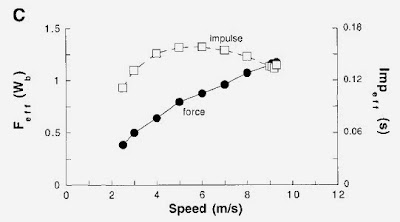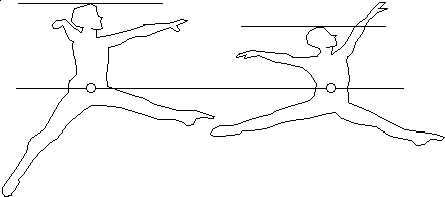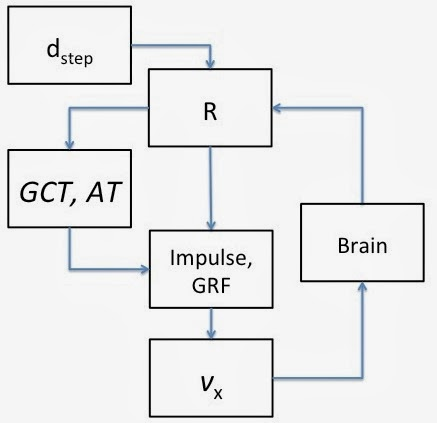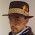## Wednesday, 15 January 2014

### Running strides part 2: Takeoff angles and other predictions

In Part 1 I showed that some basic predictions can be made about running contact times knowing only  one's turnover rate R (cadence; steps per second) as a function of vx (your forward speed in m/s) and dleg (the length of your extended leg, in metres). The first assumption I made was that R can be expressed as a function of vx and Lleg. From the early figures in part 1, this looked to be roughly true. The second assumption was that the distance of your running step, dstep, which is the distance covered per stride was equal to Lleg and that this is true regardless of your running speed.  To verify if these assumptions were indeed correct, I calculated the ground contact time (time spent on ground; GCT) and aerial time (time spent in air; AT) and compared them to actual empirical values.

Again, here are the two equations for GCT and AT, respectively:

I found my predictions were, within measurement error and natural human variation, equivalent to several published values. The next step is to then expand our horizons and make bolder predictions.

Physics of flight: aerial flight path

For any physical object, if launched at an initial angle theta and speed v the distance  covered with respect to the centre of mass (over a flat, windless surface) is supplied by the equation

Or in terms of the forward movement vx and tangential takeoff angle theta,

where g is the gravitational constant (9.81 m/s2). Although this equation assumes no air resistance, this isn't a bad assumption when comparing with studies done on treadmill, of which there are many. I did a side calculation using some air resistance modelling, and found only a 1-2% difference in distance travelled after factoring wind into the equation. Figure 1 shows the basic idea; with each running step you "launch"yourself into the air at angle theta and travel a short distance before touching ground again.Figure 1: Distance d covered by an object launched at angle theta.
Total distance covered during a step

Given we know the total distance covered per step is vx/R and that the distance covered on the ground is simply dstep (equal to Lleg), we can further estimate distance covered in each of the above phases. Equating the two sides we have

Substituting for Dtot and dair we have

Rearranging the terms Rdstep and vx as a function of takeoff angle theta,

From now on I will refer to this equation as (*).

I could not find any papers that measured the takeoff angle of the centre of mass as a function of speed. There are many studies that measure takeoff angles of specific body joints (e.g. knees, hips), but not the centre of mass itself. So I will have to analyze equation (*) indirectly. There are a reasonable number of comparisons I could make measuring this way.

Predicting the walk/run transition using (*)

It's often been a puzzler why we choose to instinctively start running past a given walking speed. But to change perspective, why do we start walking below a given running pace? Either way, the answer is around 2.5 m/s. Here I will predict one's walking speed as a function of R and dstep.

First of all, the takeoff angle theta in (*) must, on a flat surface, be greater than zero to make sense (otherwise you'd be running into the ground). You'll notice, however, it is quite possible for the bracketed term in to be less than zero. I interpret this point as the run/walk transition point. Setting the term to zero and defining this to be vwalk we have

so that

Recall that I made a loose estimate for R (which seemed to work):

Now for dstep = 0.9 m (half an average man's height), we have the quadratic equation

which yields two solutions vwalk  = 2.33 and 63 m/s, the latter extraneous. The former, however, is rather close to a realistic-looking answer. To verify this, we use it to calculate the Froude number. It is found that people naturally switch to running/walking when the Froude number (Fr) is equal to around 0.5. The Froude number is defined as

If our above prediction is correct, it should yield a value near 0.5. Plugging 2.33 m/s and Lleg = 0.9 m into our "Froude" equation we have Fr = 0.61. Not bad!

Plotting (*) for the sheer heck of it

Here's a hypothetical runner's centre of mass take off angle as a function of speed, which I think looks pretty cool on its own. (too bad there isn't much data to compare it to)Figure 2: Take-off angle at moment one leaves the ground (with respect to one's centre of mass COM)
As the runner's speed increases, it is apparent that the takeoff angle first rises, then falls. Hence your "bounciest" stride is not at your slowest pace, nor fastest, but somewhere in between. In this case the maximum bounce comes around 4 m/s, which is a medium-fast endurance running speed.

I'll now use (*) to predict impulse and ground reaction forces while running

Ground reaction forces

The force one feels during a stride (in massless G units) is the change in speed divided by the time it takes to implement this force, thus
where the +1 term is to account for standing bodyweight.

Hence GRF is now a function of forward speed, turnover, and step distance.

The impulse I is the ground contact time multiplied by the force applied, which is identical to the term we set to zero earlier:

Here is GRF - 1 and I plotted together and compared with real data:Figure 3: Contact forces and impulseFigure 4: Empirical contact forces and impulses. Data from Weyand et al.
Although the body weights are a little lower in the empirical data, the impulse estimates are very close to correct (and share the same shape). Impulse forces actually go down at faster speeds, which can be interpreted as less time to achieve a push off, hence the lower push off angle and higher turnover rate.

Predicting stair climbing with

Fro running on a non-flat surface we can include a slope "grad" (which has values usually between -1 and 1):

If grad = 0 then the surface is flat. If running uphill on a 1% slope then grad = 0.01, and so on. If I set grad = 1, then the takeoff angle is 45 degrees and we are effectively running up stairs:Figure 5: Take off angle climbing up stairs

On a slope, the air time (AT) between steps is slightly shorter be it an incline or downward slope:

Energetics of running and vertical displacement:

We can estimate at how high one's centre of mass reaches during running by transforming vertical takeoff speed and assuming it converts to potential energy:

So that

Plotting this vertical displacement as a function of speed (in cm) we have a maximum centre of mass (COM) displacement of 3.5 cm (for 6.3 m/s), and only 0.7 cm at 3 m/s. Recall this is the displacement compared with the "zero" line during takeoff.Figure 6: Maximum vertical displacement of COM from toe-off

Here is an empirical model, which shows a maximum vertical displacement of 5 cm while running at 3 m/s (regardless of surface stiffness) compared with one's standing height (your COM is negative after landing).Figure 7: Centre of mass vertical displacement at 3 m/s for two surface stiffnesses. Figure from Ferris et al.
There is a mismatch between theory and experiment; my COM displacement at 3 m/s is only 0.7 cm while theirs is measured to be much higher at 5 cm. Either way the vertical displacement is small. Why are my predictions so much lower than the measured COM values? Perhaps I could claim there's something wrong with this experiment and the model is fine.Figure 8: From The Physics of Dance. Notice how the COM moves up the torso (a few inches) as the legs move apart. It's hard to estimate COM visually.
Considering my other output parameters agree with measurement, I am suspicious there's a disagreement between measurement technique. The figure 7 diagram shows aerial phase of running lasting over 0.2 seconds. That is actually much longer than should, where AT is at most 150 ms.  No matter how you jump you'll never go above 2.7 cm vertically in 150ms total flight time. Regardless, one's centre of mass is difficult to define precisely. Their COM is defined as the middle between peak heigh at midfoot stance on landing, whereas my COM is intrinsically defined as the COM at the moment of takeoff, which could be slightly higher. Therefore at 3 m/s one might move their COM 5 cm upward/downward from that point of view.

Running efficiency and cadence:

In part 1 I used data from a study by Weland et al titled "Faster top running speeds are achieved with greater ground forces not more rapid leg movements." That study, and a conversation with Alex Hutchinson on the subject got me thinking about efficiency. That study implies that turnover rates peaked for a runner too early, they could not run any faster.

What if we compared a group of runners all of equal height and weight on a treadmill for running efficiency. My hypothesis: for a fast but non-sprinting speed V (say 5-8 m/s), if runner A has a lower cadence than runner B at that speed, then runner A is also more efficient than runner B. Efficiency is measures as oxygen consumed per meter per kilogram body weight. Cadence is measured as steps per minute.

Energy cost of running ~ Cadence

Although I could not compare any papers directly using this idea, I did come across this paper via Sweat Science that measured running economy versus takeoff angle. It may be a bit of a stretch, but decided to create figure 9 by varying R for an imagined group of runners all running at the same speed but with different cadences. One cannot claim a direct comparison between figure 9 and 10, but if cadence is linked with running economy then "bouncy" strides are predicted to be more efficient. (NB: I had originally thought the takeoff angles in figure 10 were too low, until I found my own angle calculation were just as small).Figure 9: Cadence vs takeoff angleFigure 10: Running economy vs stride angle. From Concejero et al

Conclusions

This two-part post was intended to see what minimum information could tell you as much as possible about your running. It turns out knowing cadence R as a function of speed (and your leg length) tells you a lot, including ground contact time, aerial time, impulse forces, landing impact forces, and perhaps even predicts running economy.

Although R can be directly related to running speed, i.e. R = f(vx), speed is really the result of cadence (plus a whole lot of other things that are harder to quantify). That is to say, we'd expect that vx = f(R). The true answer is cyclical: your brain is aware that you are running a constant speed and controls both speed and cadence in a feedback loop (If not, it would be much harder than it is to keep a constant pace). The following diagram summarizes how we can organize the cyclical tree of running information.Figure 11: Simplified flow chart of running. Speed is the final product, but it feeds back into the brain's control of R, the main control of speed and a host of other measures. Dstep = distance covered while on ground, R is cadence (steps per second), GCT = Ground contact time, AT = Aerial time, GRF = Ground reaction force, Impulse = force time contact time, vx = forward running speed.
Certain seemingly complex values such as GRF and stride distance can be accurately determined using only a few input parameters. I haven't been able to derive energy of running, but this is good enough for now. No grand conclusions here, just some observations I was looking finally share.

1.2.